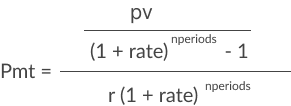# Pmt

The Pmt function returns the periodic payment for an investment.

You can use it to calculate constant payments for an investment or a loan, based on the loan amount, number of periods, and constant interest rate.

Pmt is part of the set of financial functions that Sigma supports.

## Syntax

`PMT(rate, nperiods, pv, [fv], [type])`

The Pmt function has the following arguments:

rate
Required.
The interest rate for the loan.
nperiods
Required.
The total number of payments for the loan.
pv
Required.
The present value, or total value of all loan payments; the amount borrowed.
fv
Optional.
The future value, or a cash balance you want after the last payment is made.
Defaults to 0 (zero).
type
Optional.
When payments are due:
0
End of period
1
Beginning of period
Default is 0.

The general formula for the Pmt function is:## Notes

• Be consistent with the units for rate and nperiods arguments. If you make monthly payments on a two-year loan at an annual interest rate of 7%, use the rate calculation of 0.07/12 and nperiods calculation of 2*12. For annual payments on the same loan, use the rate of 0.07 and nperiods of 2.
• Pmt returns the payment calculation that includes both the principal and interest portions. It does not include taxes, reserve payments, or fees.
• To calculate the total amount paid over the duration of the loan, multiply the returned Pmt value by nperiods.

## Examples

`Pmt(.07/12,2*12,10000)`

The monthly payment for a loan of 10,000, with an annual interest rate of 7% is \$447.73.

`Pmt(.07,2,10000)`

The annual payment for a loan of 10,000, with an annual interest rate of 7% is \$5,530.92.